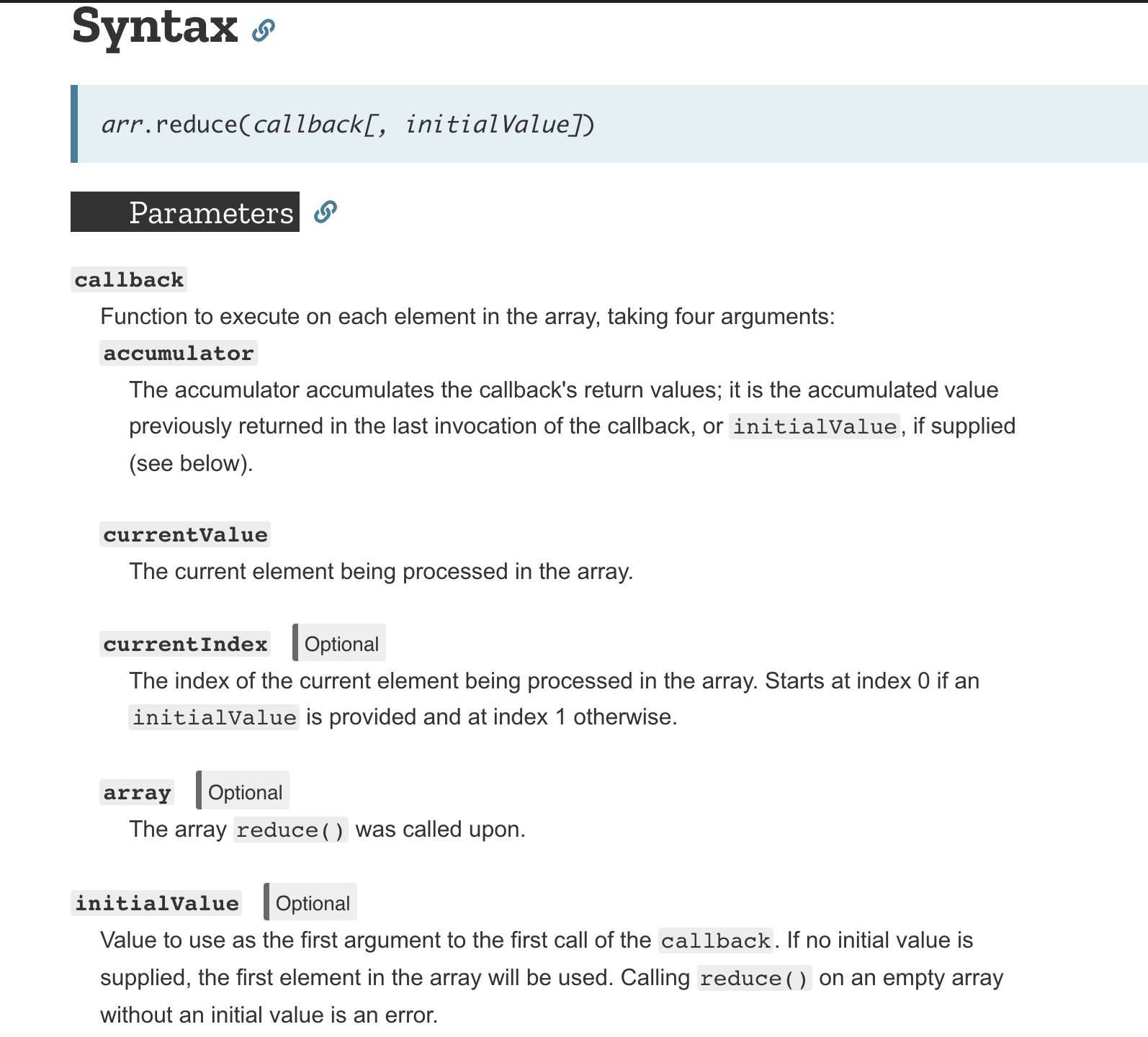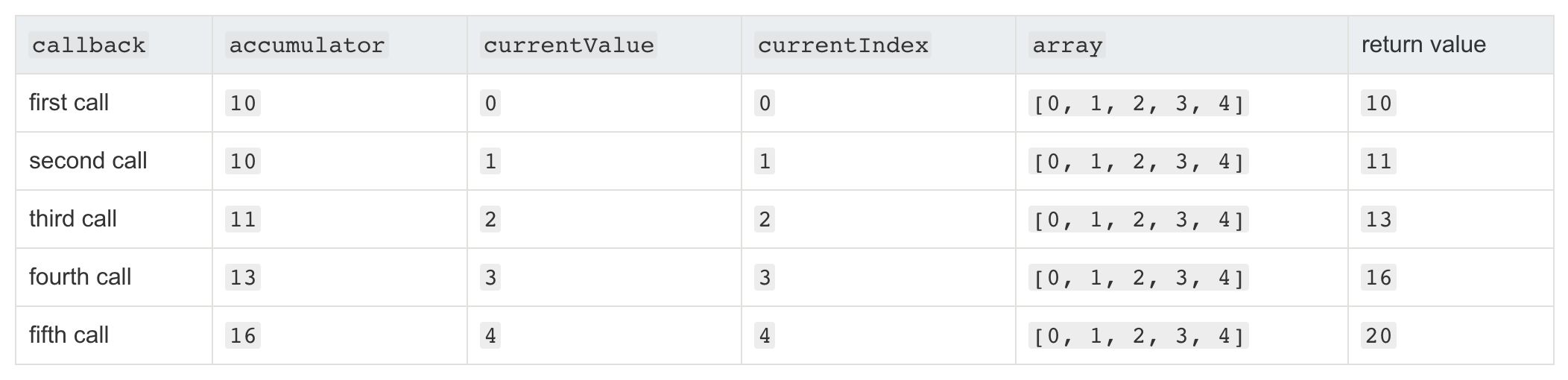In this section we are picking up what is left from Part1initialValue and currentIndex parameter in .reduce(), as well as some of .reduce()’s application.Fig. 1; from MDN

Regarding whether the initial value is set, there is connection between initialValue and the start point of currentIndex (see Fig. 1 “currentIndex”), though not fully manipulatable:

``````let arr = [0, 1, 2, 3, 4];
function getSum(total, num) {
}
// Fig. 2
console.log(
arr.reduce(getSum)
);
// Fig. 3
console.log(
arr.reduce(getSum, 10)
);
``````Fig. 2; from MDNFig. 3; from MDN

When .reduce() is called without an initialValue, currentValue is set to go from “index(currentIndex) 1 “  (arr; 1 in our example) in the first rotation of the loop,  where the one that is set with an initialValue “10” is going from “index(currentIndex) 0” (arr; 0) in the first call.

Aside from adding up the values in a plain array of numbers, .reduce() can also be used to

``````let data = [
{
country: 'China',
population: 1409517397,
},
{
country: 'India',
population: 1339180127,
},
{
country: 'USA',
population: 324459463,
},
{
country: 'Indonesia',
population: 263991379,
}
];

let sum = data.reduce(function (accumulator, data) {
return accumulator + data.population;
}, 0); // set initValue 0 to make sum a number instead of a string

console.log(sum); // should output 3337148366
``````
``````let names = ['Alice', 'Bob', 'Tiff', 'Bruce', 'Alice'];
let countedNames = names.reduce(function (allNames, name) {
if (name in allNames) {
allNames[name]++;
}
else {
allNames[name] = 1;
}
return allNames;
}, {});
// countedNames is:
// { 'Alice': 2, 'Bob': 1, 'Tiff': 1, 'Bruce': 1 }
``````

…more application of `.reduce()` can be found on MDN